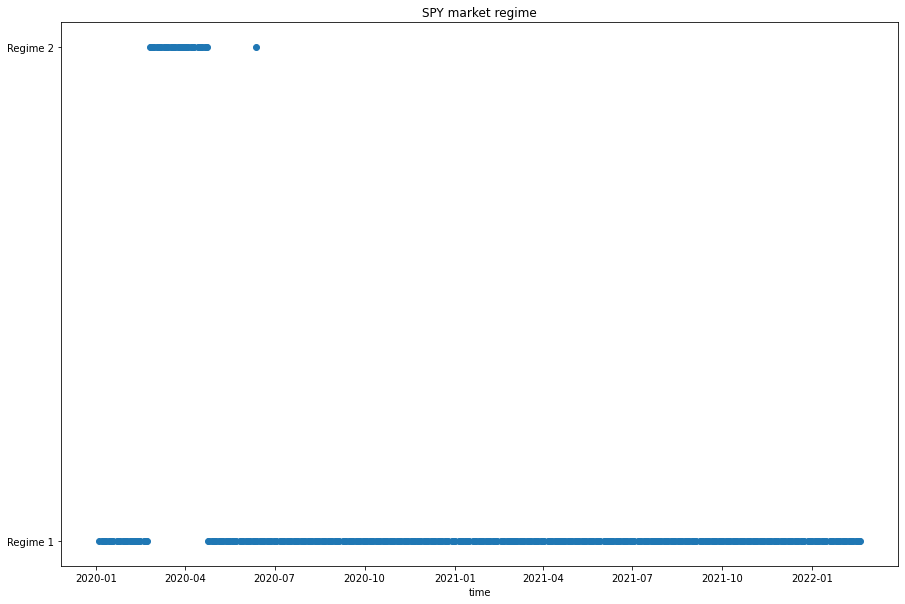# Machine Learning

## Hmmlearn

### Introduction

This page explains how to build, train, test, and store Hmmlearn models.

### Import Libraries

Import the Hmmlearn library.

from hmmlearn import hmm
import joblib

You need the joblib library to store models.

### Get Historical Data

Get some historical market data to train and test the model. For example, to get data for the SPY ETF during 2020 and 2021, run:

qb = QuantBook()
history = qb.History(symbol, datetime(2020, 1, 1), datetime(2022, 1, 1)).loc[symbol]

### Prepare Data

You need some historical data to prepare the data for the model. If you have historical data, manipulate it to train and test the model. Follow these steps to prepare the data:

1. Select the close column of the historical data DataFrame.
2. closes = history['close']
3. Call the pct_change method and then drop the first row.
4. daily_returns = closes.pct_change().iloc[1:]
5. Call the reshape method.
6. X = daily_returns.values.reshape(-1, 1)

### Train Models

You need to prepare the historical data for training before you train the model. If you have prepared the data, build and train the model. In this example, assume the market has only 2 regimes and the market returns follow a Gaussian distribution. Therefore, create a 2-component Hidden Markov Model with Gaussian emissions, which is equivalent to a Gaussian mixture model with 2 means. Follow these steps to create the model:

1. Call the GaussianHMM constructor with the number of components, a covariance type, and the number of iterations.
2. model = hmm.GaussianHMM(n_components=2, covariance_type="full", n_iter=100)
3. Call the fit method with the training data.
4. model.fit(X)

### Test Models

You need to build and train the model before you test its performance. If you have trained the model, test it on the out-of-sample data. Follow these steps to test the model:

1. Call the predict method with the testing dataset.
2. y = model.predict(X)
3. Plot the regimes in a scatter plot.
4. plt.figure(figsize=(15, 10))
plt.scatter(ret.index, [f'Regime {n+1}' for n in y])
plt.title(f'{symbol} market regime')
plt.xlabel("time")
plt.show()### Store Models

You can save and load Hmmlearn models using the Object Store.

#### Save Models

Follow these steps to save models in the Object Store:

1. Set the key name of the model to be stored in the Object Store.
2. model_key = "model"
3. Call the GetFilePath method with the key.
4. file_name = qb.ObjectStore.GetFilePath(model_key)

This method returns the file path where the model will be stored.

5. Call the dump method with the model and file path.
6. joblib.dump(model, file_name)

If you dump the model using the joblib module before you save the model, you don't need to retrain the model.

You must save a model into the Object Store before you can load it from the Object Store. If you saved a model, follow these steps to load it:

1. Call the ContainsKey method.
2. qb.ObjectStore.ContainsKey(model_key)

This method returns a boolean that represents if the model_key is in the Object Store. If the Object Store does not contain the model_key, save the model using the model_key before you proceed.

3. Call the GetFilePath method with the key.
4. file_name = qb.ObjectStore.GetFilePath(model_key)

This method returns the path where the model is stored.

5. Call the load method with the file path.
6. loaded_model = joblib.load(file_name)

This method returns the saved model.

You can also see our Videos. You can also get in touch with us via Discord.